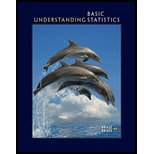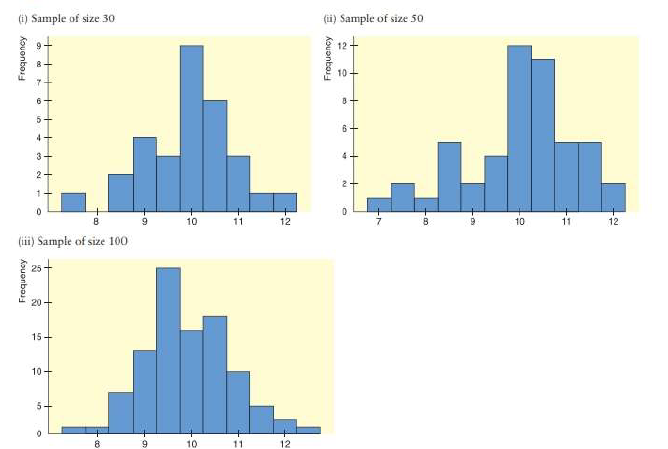Chapter 2.1, Problem 11PUnderstanding Basic Statistics

8th Edition
Charles Henry Brase + 1 other
ISBN: 9781337558075

Solutions

Chapter
SectionUnderstanding Basic Statistics

8th Edition
Charles Henry Brase + 1 other
ISBN: 9781337558075
Textbook Problem

Interpretation Histograms of random sample data are often used as an indication of the shape of the underlying population distribution. The histograms on the next page are based on random sample of size 30.50, and 100 from the same population.(a) Using the midpoint labels of the three histograms, what would you say about the estimated range of the population data from smallest to largest? Does the balk of the data seem to be between 8and 12 in all three histograms?(b) The population distribution from which the samples were draws is symmetric and mound-shaped, with the top of the mound at10.95% of the data between 8 and 12, and 99.7% of data between 7 and 13. How well does each histogram reflect these characteristics?(a)

To determine

The range of the population data from smallest to largest and whether the bulk of the data seem to be between 8 and 12 in all three histograms.

Explanation

Using the midpoint labels of the three histograms, the range of the population data from smallest to largest is shown in the following table:

 Smallest Largest Histogram (i) 8 12 Histogram (ii) 7 12 Histogram (iii) 7 13

In the first histogram, the data fall between 8 and 12 but few values fall outside 8...

(b)

To determine

To explain: Whether the population distribution of three histograms was symmetric and mound-shaped.

Still sussing out bartleby?

Check out a sample textbook solution.

See a sample solution

The Solution to Your Study Problems

Bartleby provides explanations to thousands of textbook problems written by our experts, many with advanced degrees!

Get Started

In Exercises 516, evaluate the given quantity. log5125

Finite Mathematics and Applied Calculus (MindTap Course List)

Prove that limx0x2cos(1/x2)=0.

Single Variable Calculus: Early Transcendentals, Volume I

16. If , find the following. (a) (b) (c)

Mathematical Applications for the Management, Life, and Social Sciences

Factor completely: 15y2y6

Elementary Technical Mathematics

Evaluate the integral. 8. t2sintdt

Single Variable Calculus: Early Transcendentals

In O,OY=5 and XZ=6. If XWWY, find WZ.

Elementary Geometry for College Students

In Exercises 15-28, construct a truth table for the compound proposition. (pq)(qp)

Finite Mathematics for the Managerial, Life, and Social Sciences

2 1 0 does not exist

Study Guide for Stewart's Multivariable Calculus, 8th

Using the power series for cos x, the sum of the series is: cos(0.0625) cos(0.25) cos(0.5)

Study Guide for Stewart's Single Variable Calculus: Early Transcendentals, 8th